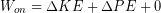# 10.11: Comparing Work-Energy and Energy Conservation*

$$\newcommand{\vecs}{\overset { \rightharpoonup} {\mathbf{#1}} }$$ $$\newcommand{\vecd}{\overset{-\!-\!\rightharpoonup}{\vphantom{a}\smash {#1}}}$$$$\newcommand{\id}{\mathrm{id}}$$ $$\newcommand{\Span}{\mathrm{span}}$$ $$\newcommand{\kernel}{\mathrm{null}\,}$$ $$\newcommand{\range}{\mathrm{range}\,}$$ $$\newcommand{\RealPart}{\mathrm{Re}}$$ $$\newcommand{\ImaginaryPart}{\mathrm{Im}}$$ $$\newcommand{\Argument}{\mathrm{Arg}}$$ $$\newcommand{\norm}{\| #1 \|}$$ $$\newcommand{\inner}{\langle #1, #2 \rangle}$$ $$\newcommand{\Span}{\mathrm{span}}$$ $$\newcommand{\id}{\mathrm{id}}$$ $$\newcommand{\Span}{\mathrm{span}}$$ $$\newcommand{\kernel}{\mathrm{null}\,}$$ $$\newcommand{\range}{\mathrm{range}\,}$$ $$\newcommand{\RealPart}{\mathrm{Re}}$$ $$\newcommand{\ImaginaryPart}{\mathrm{Im}}$$ $$\newcommand{\Argument}{\mathrm{Arg}}$$ $$\newcommand{\norm}{\| #1 \|}$$ $$\newcommand{\inner}{\langle #1, #2 \rangle}$$ $$\newcommand{\Span}{\mathrm{span}}$$$$\newcommand{\AA}{\unicode[.8,0]{x212B}}$$

In the previous chapters we analyzed a person’s jump using the work-energy principle rather than the Law of Conservation of Energy. Examining that correspondence between these concepts will allow us to learn a few important concepts. Let’s refresh ourselves with that example:

## Everyday Examples: Jumping

During a jump a person’s legs might apply a force of 1200 N upward on their center of mass while the center of mass moves 0.3 m upward. Let’s figure out what their launch speed and hang time will be if the person has a weight of 825 N.

First we calculate the work done by their legs.Gravity was acting on them during the launch phase as well, so we need to calculate the work done by gravity:The net work is then:The work-energy principle tells us to set the change in kinetic energy equal to the net work. We will keep in mind that they started at rest, so the initial kinetic energy was zero.We can see that we need the person’s mass. We just divide their weight by g= 9.8 m/s/s to find it:We isolate the final speed at the end of the launch phase (as the person leaves the ground) and insert the mass.Then we take the square root the result to find the speed:In the first part of the jumping example we calculated the net work on the object and used the work-energy principle to find the change in kinetic energy. In equation form it looks like this:Moving the work done by gravity to the other side:The Law of Conservation of Energy looks like this:Remembering that we ignored friction and air resistance, and that nothing was permanently deformed,.Gravity did negative work because it points down and motion was upward, but the effect of that work was to increase potential energy by transferring some of the person’s kinetic energy into their owngravitational potential energy. Therefore change in gravitational potential energy should be the negative of the work done by gravity. This work was internal to the Earth-person system, so the only work being done on the person’s center of gravity from the outside was work done by the legs. Making these replacements we have:Which is exactly the work-energy principle we started with. We can either use the work-energy principle on a given object and include work done by resistance to compression and gravity in the net work, and say all of that work contributes to changing kinetic energy, or instead use the Law of Conservation of Energy and instead say that work done by elastic forces and gravity contributes to elastic potential energy and gravitational potential energy instead of kinetic energy. Either way is equivalent, as we have just seen.

This page titled 10.11: Comparing Work-Energy and Energy Conservation* is shared under a CC BY-NC-SA 4.0 license and was authored, remixed, and/or curated by Lawrence Davis (OpenOregon) via source content that was edited to the style and standards of the LibreTexts platform; a detailed edit history is available upon request.